## for Blind Inverse Problems

A block-coordinate PnP method with theoretical analysis for blind inverse problems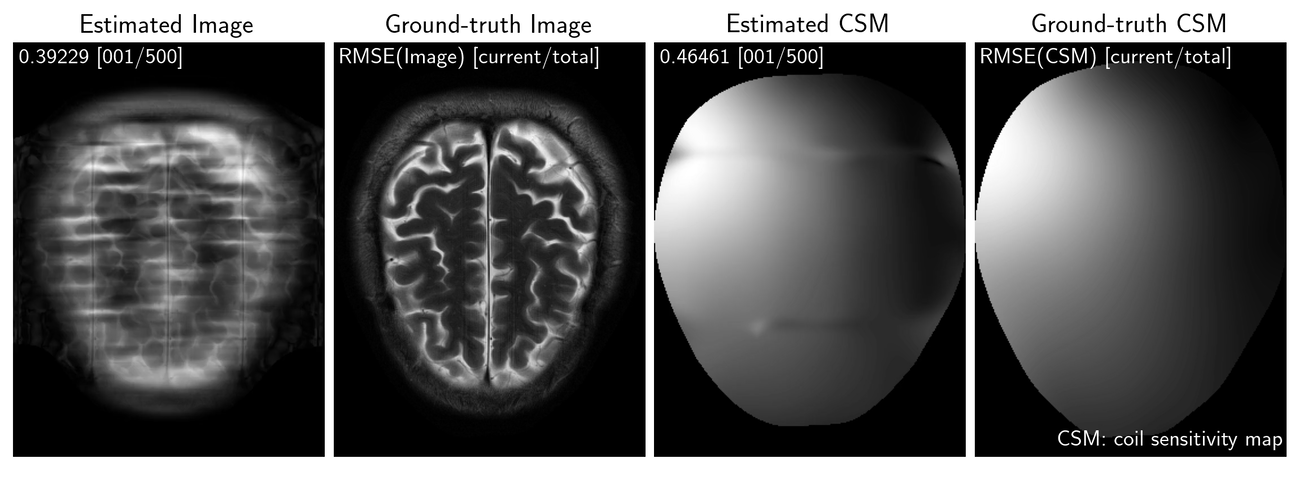Figure 1: Evolution of the estimates provided by BC-PnP on compressive sensing parallel magnetic resonance imaging (CS-PMRI). Note how BC-PnP can provide high quality estimates of both the MR image and the coil sensitivity map.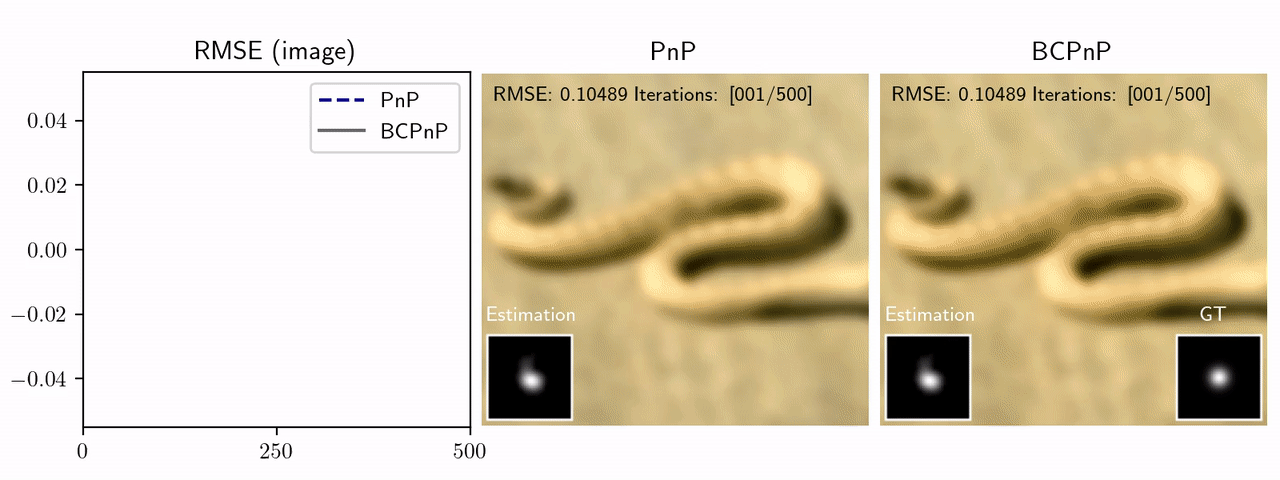Figure 2: Evolution of the estimates provided by BC-PnP and the traditional PnP that uses a pre-estimated blur kernel on blind image deblurring. Note how by jointly estimating the image and the blur kernel, BC-PnP performs better than the traditional PnP.

## Abstract

Plug-and-play (PnP) prior is a well-known class of methods for solving imaging inverse problems by computing fixed-points of operators combining physical measurement models and learned image denoisers. While PnP methods have been extensively used for image recovery with known measurement operators, there is little work on PnP for solving blind inverse problems. We address this gap by presenting a new block-coordinate PnP (BC-PnP) method that efficiently solves this joint estimation problem by introducing learned denoisers as priors on both the unknown image and the unknown measurement operator. We present a new convergence theory for BC-PnP compatible with blind inverse problems by considering nonconvex data-fidelity terms and expansive denoisers. Our theory analyzes the convergence of BC-PnP to a stationary point of an implicit function associated with an approximate minimum mean-squared error (MMSE) denoiser. We numerically validate our method on two blind inverse problems: automatic coil sensitivity estimation in magnetic resonance imaging (MRI) and blind image deblurring. Our results show that BC-PnP provides an efficient and principled framework for using denoisers as PnP priors for jointly estimating measurement operators and images.

## Convergence Behaviour of BC-PnP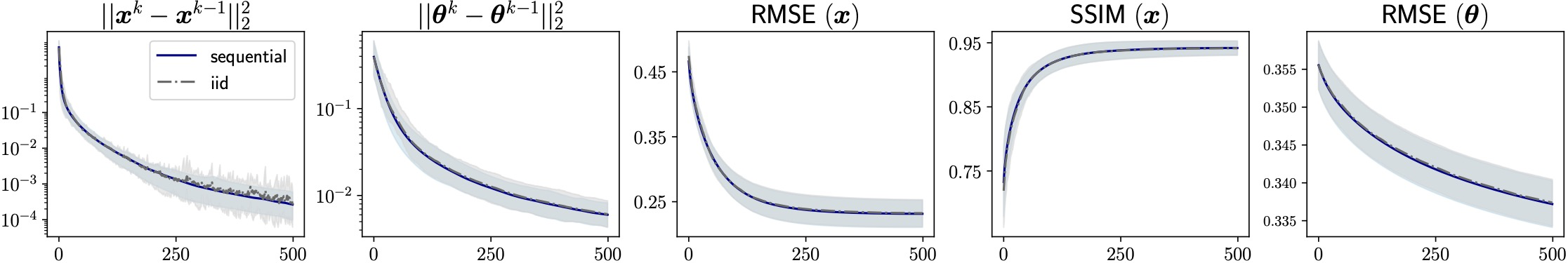Figure 3: Illustration of the BC-PnP convergence using the sequential and random i.i.d. block selection rules on CS-PMRI with the sampling factor R = 8. Leftmost two plots: Evolution of the distance between two consecutive image and CSM iterates. Rightmost three plots: Evolution of the RMSE and SSIM metrics relative to the true solutions across BC-PnP iterations. Note how both block selection rules lead to a nearly identical convergence behaviour of BC-PnP in this experiment.

## BC-PnP for compressed sensing parallel MRI (CS-PMRI) with automatic coil sensitivity calibration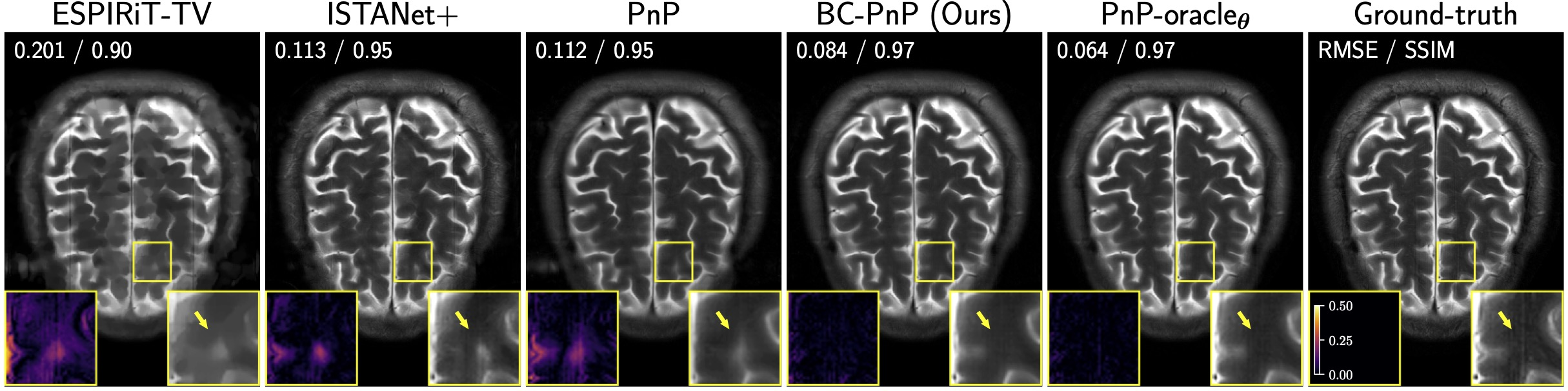Figure 4: Illustration of results from several well-known methods on CS-PMRI with the sampling factor R = 6. The quantities in the top-left corner of each image provide RMSE and SSIM values for each method. The squares at the bottom of each image visualize the error and the corresponding zoomed area in the image. Note how BC-PnP using a deep denoiser on the unknown CSMs outperforms uncalibrated PnP and matches PnP-oracleθ that knows the true CSMs.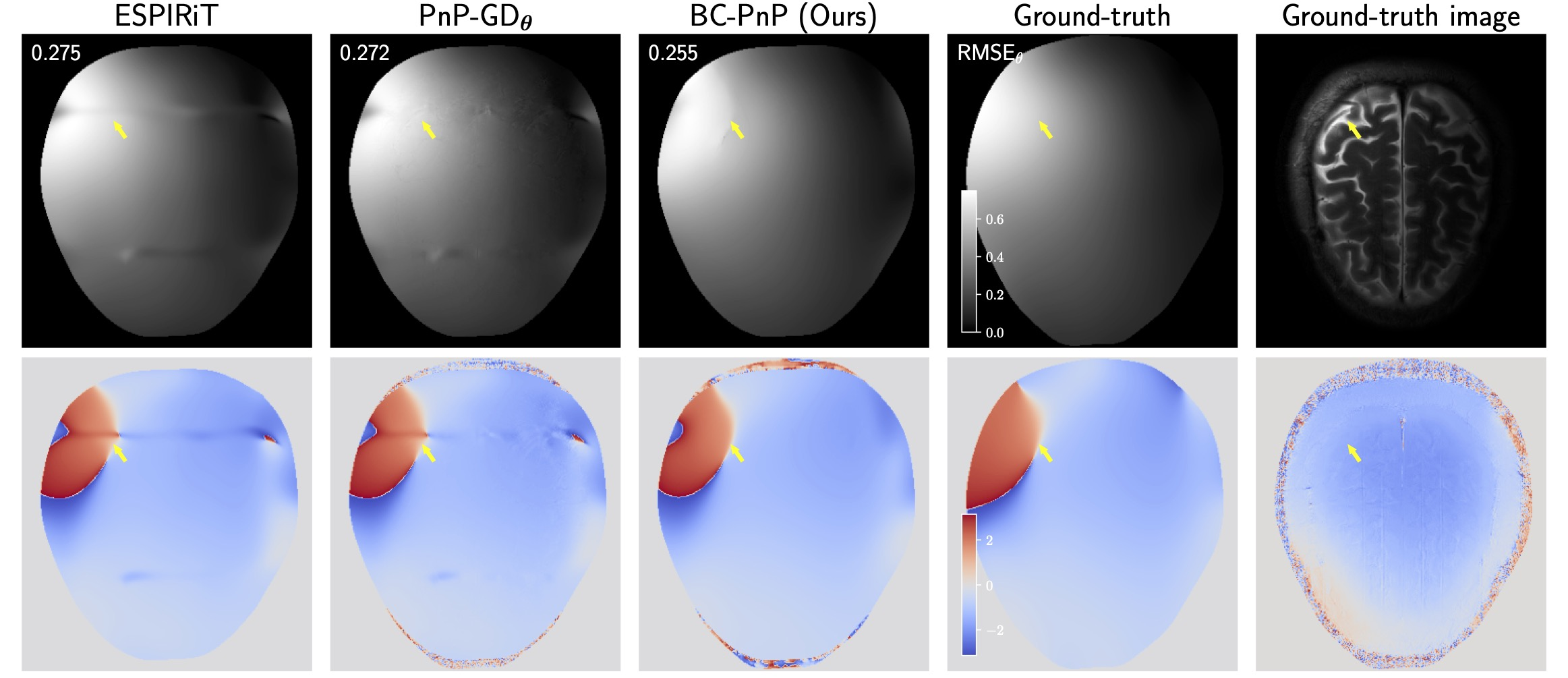Figure 5: Illustration of estimated CSM from several methods on CS-PMRI with the sampling factor R = 6. The top and the bottom rows are the magnitude and the phase of the CSMs, respectively. The quantities in the top-left corner of each image in the top row provide RMSE values for each method. Ground-truth image was obtained using the fully sampled data corresponding to the ground truth CSMs. This figure highlights the effectiveness of BC-PnP for estimating the measurement operator.

## BC-PnP for blind image deblurring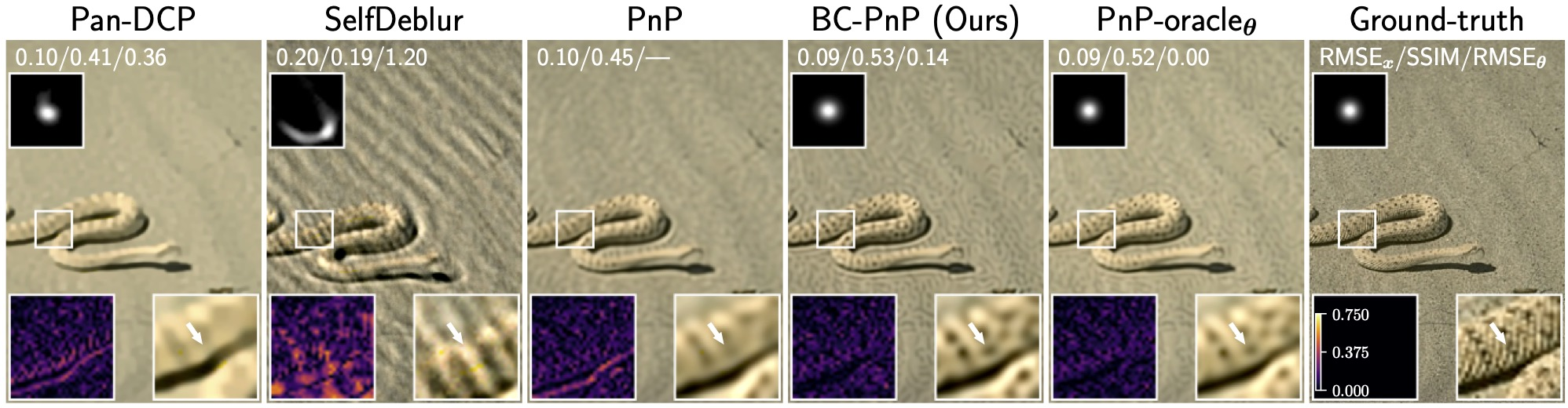Figure 6: Illustration of results from several well-known methods on blind image deblurring with the Gaussian kernel. The squares at the top of each image show the estimated kernels. The quantities in the top-left corner of each image provide RMSE and SSIM values for each method. The squares at the bottom of each image highlight the error and the corresponding zoomed image region. Note how the BC-PnP using a deep denoiser on the unknown kernel significantly outperforms the traditional PnP method and matches the performance of the oracle PnP method that knows the true blur kernel. Note also the effectiveness of BC-PnP for estimating the unknown blur kernel.

## Bibtex

@article{Gan.etal2023, author={Gan, Weijie and Shoushtari, Shirin and Hu, Yuyang, and Liu, Jiaming, and An, Hongyu and Kamilov, Ulugbek S.}, title={Block Coordinate Plug-and-Play Methods for Blind Inverse Problems}, note={arXiv:2305.12672}, year={2023} }